# RD Sharma Solutions for Class 10 Maths Chapter 4 Triangles Exercise 4.3

The similarity of triangles is an amazing chapter to learn about. From this exercise, students will learn about the internal and external bisectors of an angle of a triangle. The chapter-wise RD Sharma Solutions Class 10 developed by experts at BYJU’S is a very useful tool for students to refer and prepare confidently for their exams. Students can also download RD Sharma Solutions for Class 10 Maths Chapter 4 Triangles Exercise 4.3 PDF provided below.

## RD Sharma Solutions for Class 10 Maths Chapter 4 Triangles Exercise 4.3 Download PDF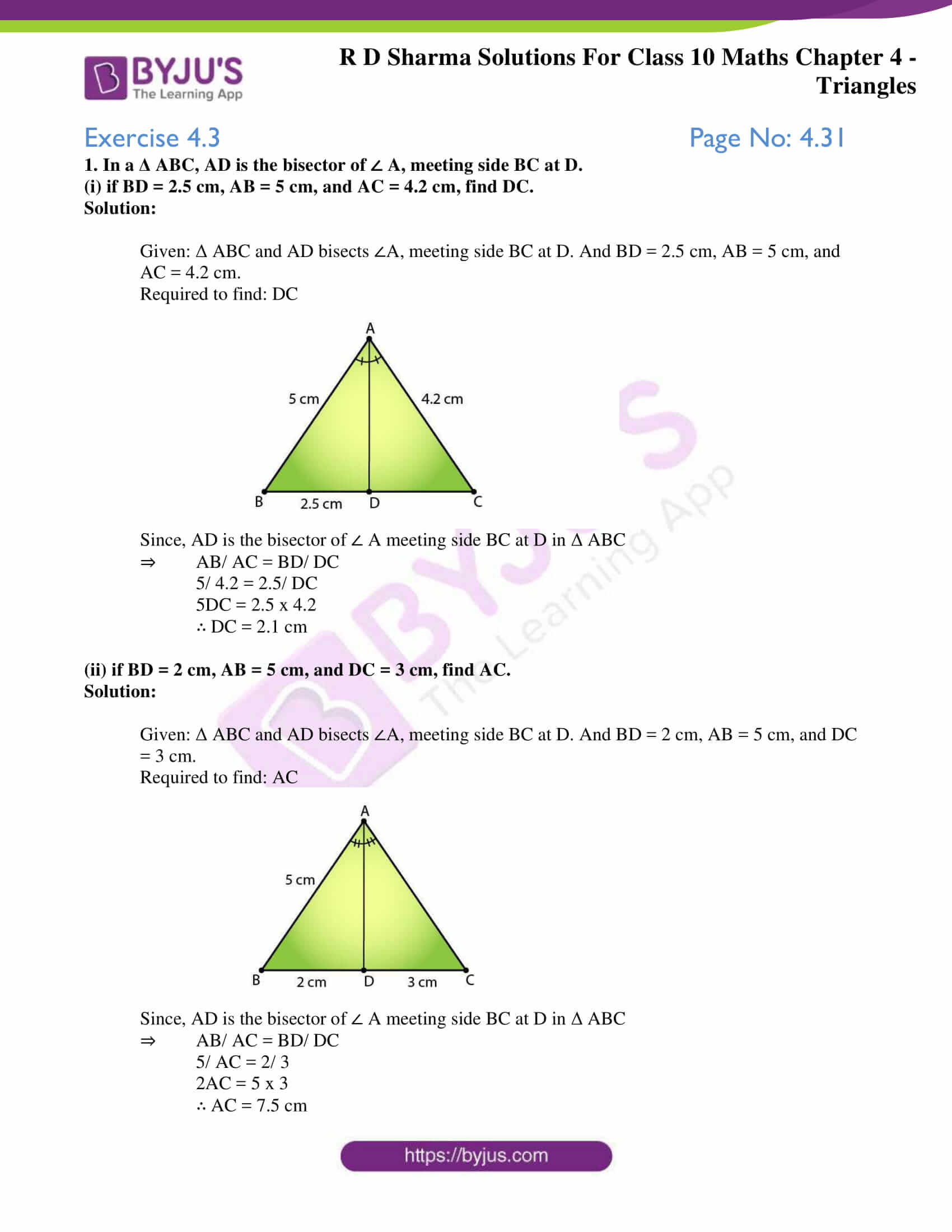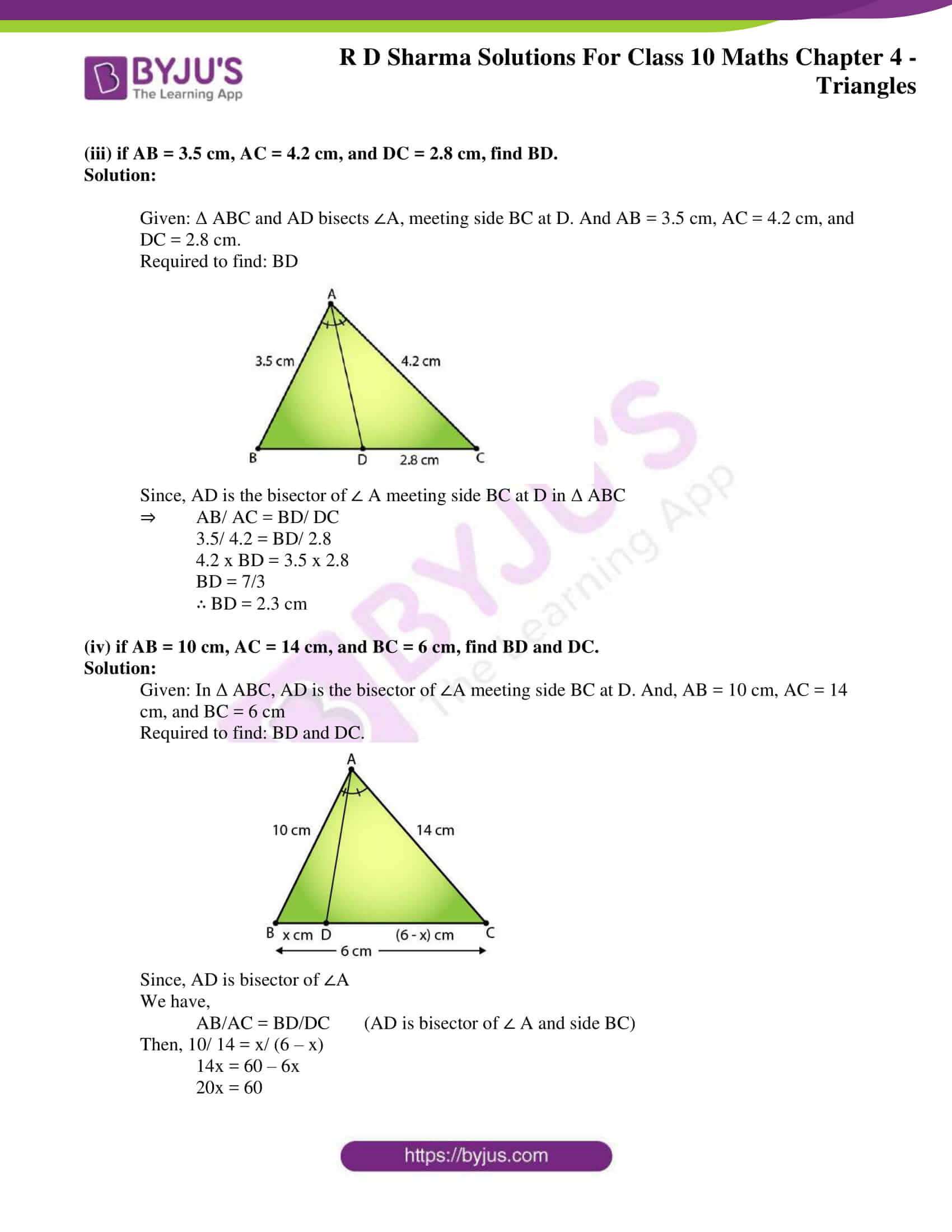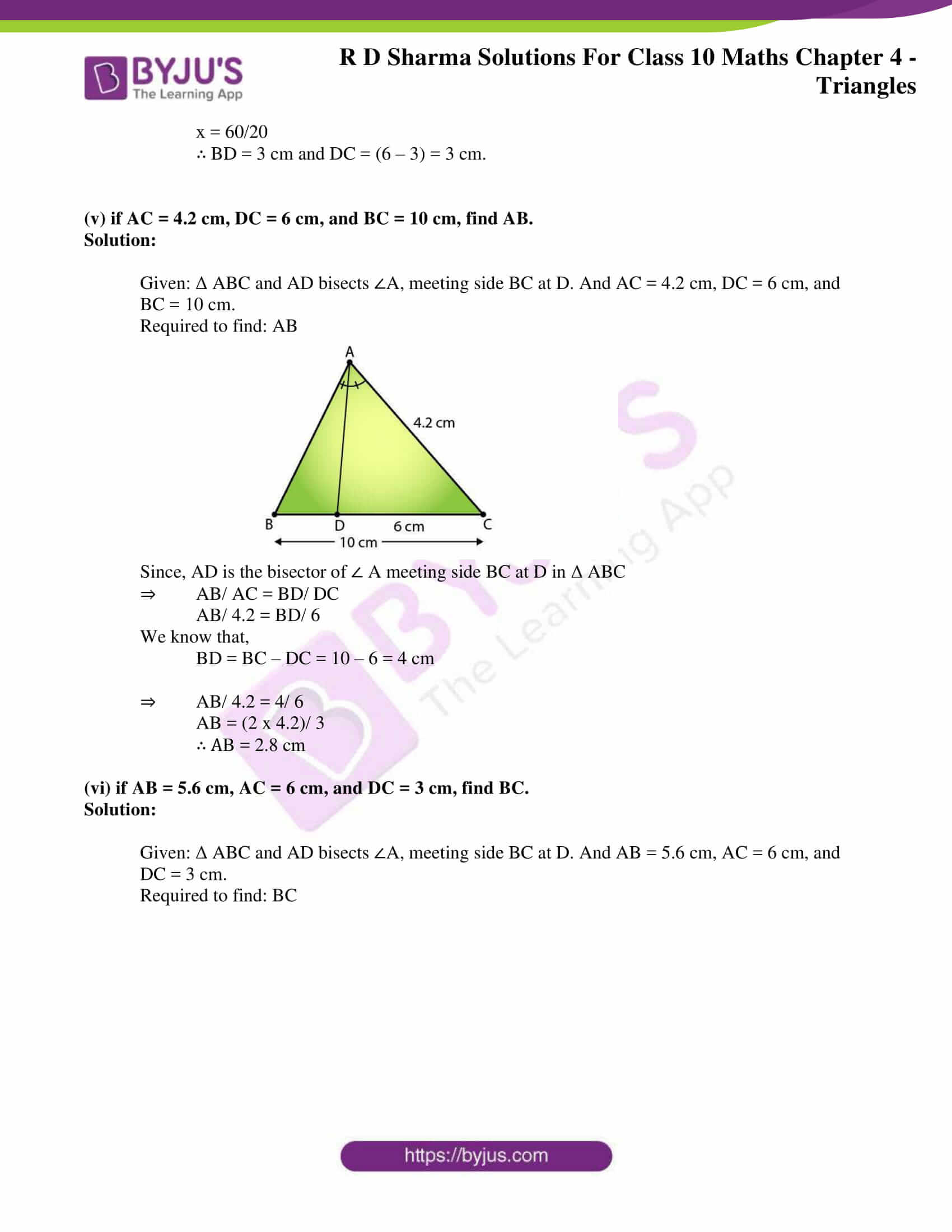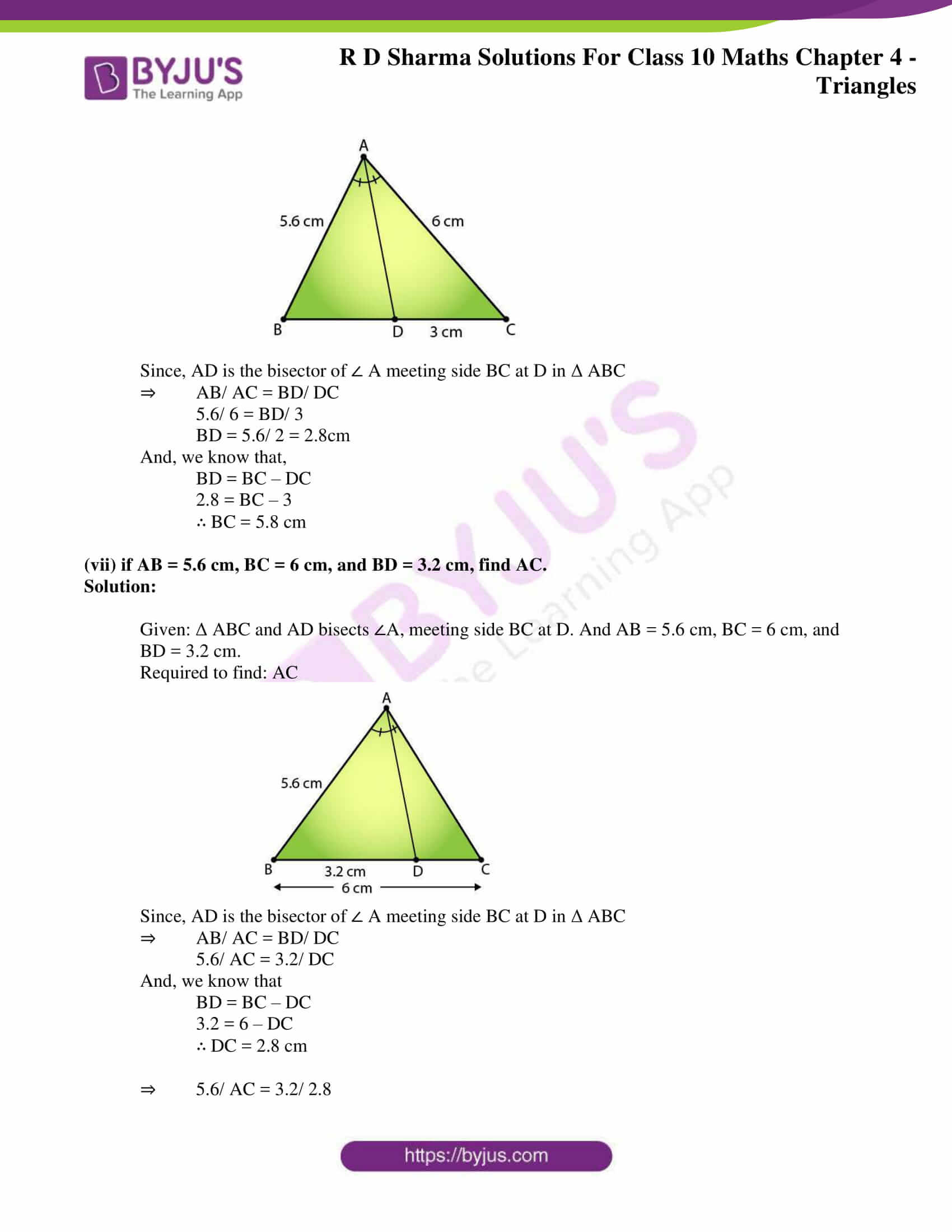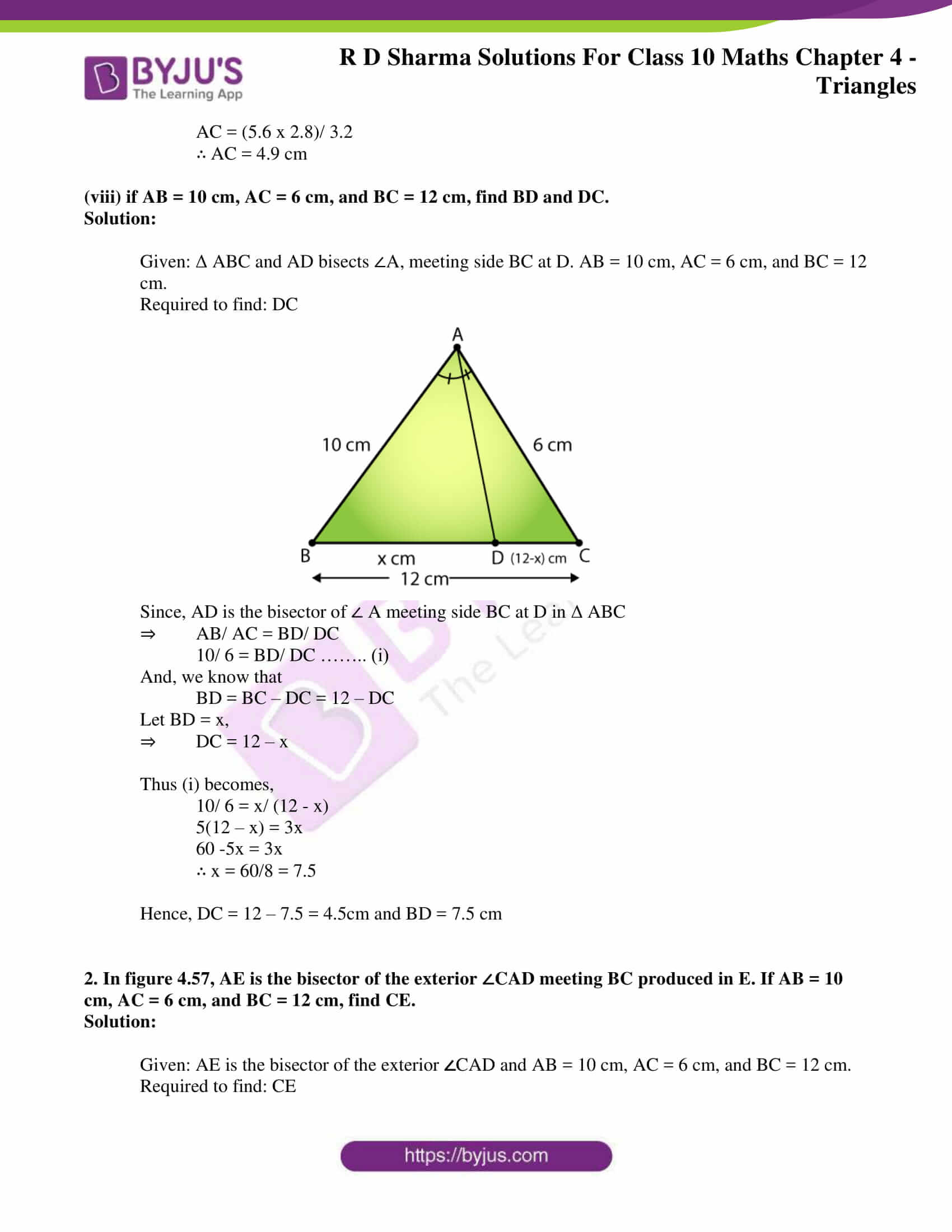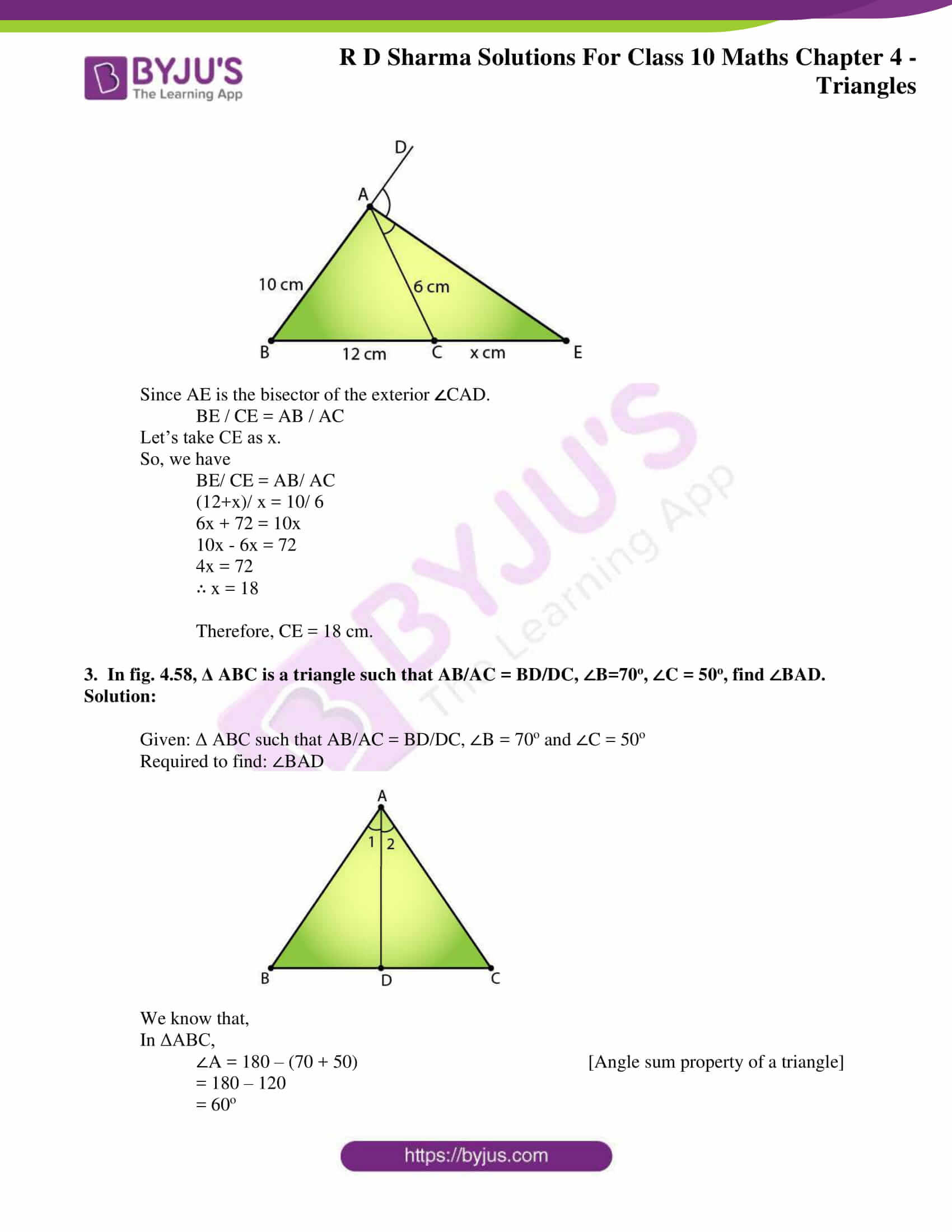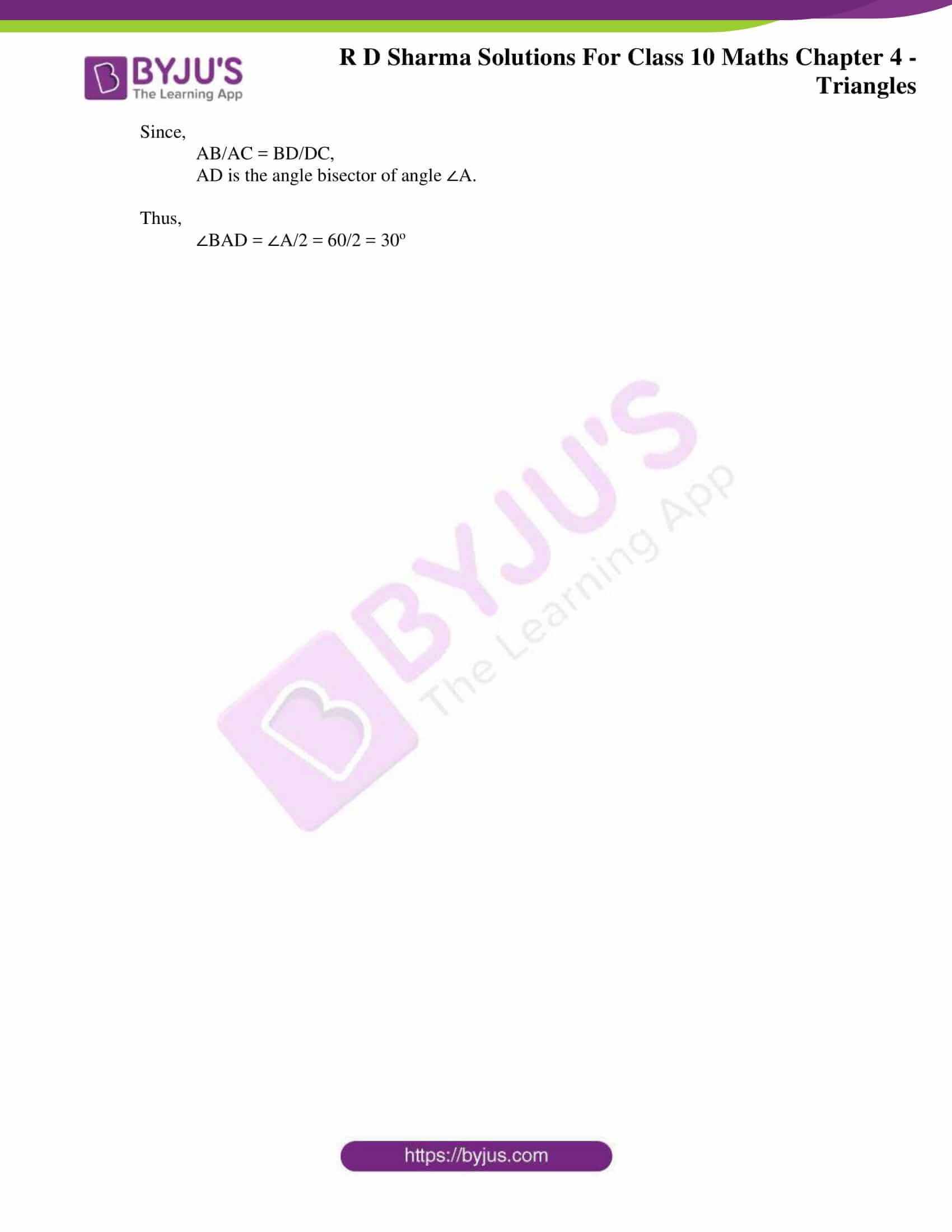### Access RD Sharma Solutions for Class 10 Maths Chapter 4 Triangles Exercise 4.3

1. In a Δ ABC, AD is the bisector of ∠ A, meeting side BC at D.

(i) if BD = 2.5 cm, AB = 5 cm, and AC = 4.2 cm, find DC.

Solution:

Given: Δ ABC and AD bisects ∠A, meeting side BC at D. And BD = 2.5 cm, AB = 5 cm, and AC = 4.2 cm.

Required to find: DC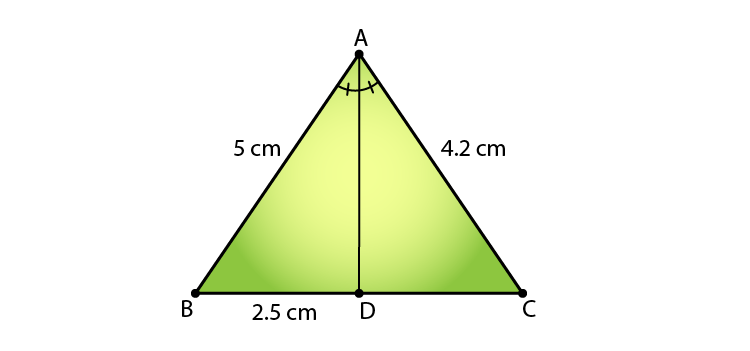Since, AD is the bisector of ∠ A meeting side BC at D in Δ ABC

⇒ AB/ AC = BD/ DC

5/ 4.2 = 2.5/ DC

5DC = 2.5 x 4.2

∴ DC = 2.1 cm

(ii) if BD = 2 cm, AB = 5 cm, and DC = 3 cm, find AC.

Solution:

Given: Δ ABC and AD bisects ∠A, meeting side BC at D. And BD = 2 cm, AB = 5 cm, and DC = 3 cm.

Required to find: AC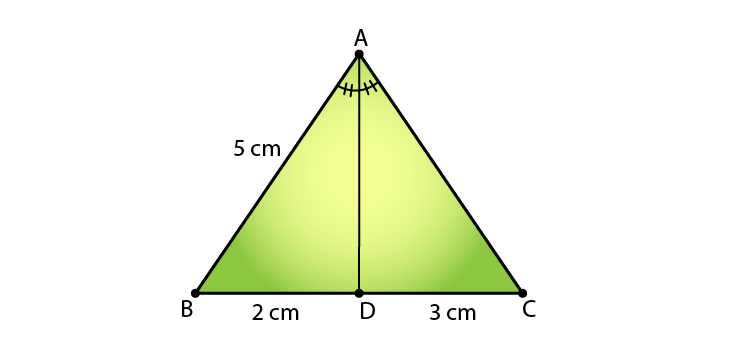Since, AD is the bisector of ∠ A meeting side BC at D in Δ ABC

⇒ AB/ AC = BD/ DC

5/ AC = 2/ 3

2AC = 5 x 3

∴ AC = 7.5 cm

(iii) if AB = 3.5 cm, AC = 4.2 cm, and DC = 2.8 cm, find BD.

Solution:

Given: Δ ABC and AD bisects ∠A, meeting side BC at D. And AB = 3.5 cm, AC = 4.2 cm, and DC = 2.8 cm.

Required to find: BD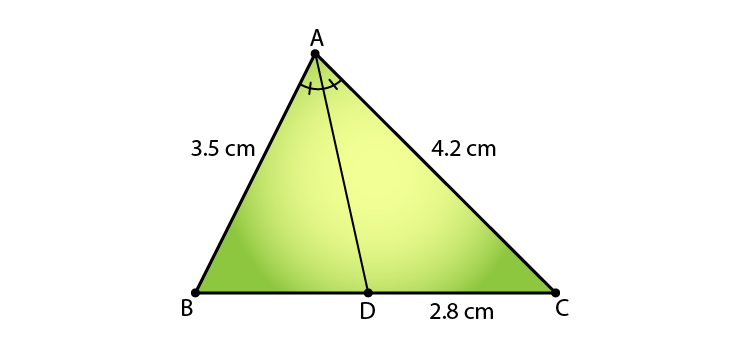Since, AD is the bisector of ∠ A meeting side BC at D in Δ ABC

⇒ AB/ AC = BD/ DC

3.5/ 4.2 = BD/ 2.8

4.2 x BD = 3.5 x 2.8

BD = 7/3

∴ BD = 2.3 cm

(iv) if AB = 10 cm, AC = 14 cm, and BC = 6 cm, find BD and DC.

Solution:

Given: In Δ ABC, AD is the bisector of ∠A meeting side BC at D. And, AB = 10 cm, AC = 14 cm, and BC = 6 cm

Required to find: BD and DC.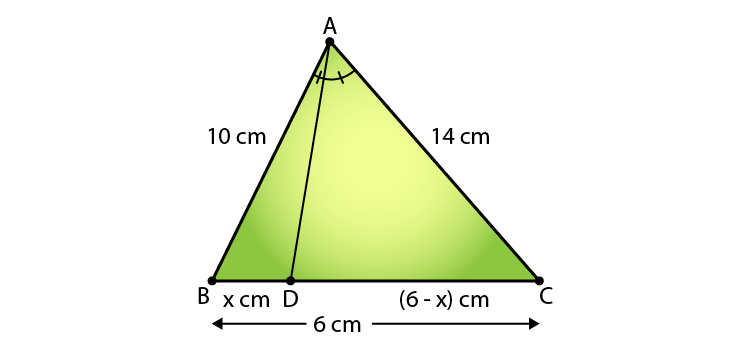Since, AD is bisector of ∠A

We have,

AB/AC = BD/DC (AD is bisector of ∠ A and side BC)

Then, 10/ 14 = x/ (6 – x)

14x = 60 – 6x

20x = 60

x = 60/20

∴ BD = 3 cm and DC = (6 – 3) = 3 cm.

(v) if AC = 4.2 cm, DC = 6 cm, and BC = 10 cm, find AB.

Solution:

Given: Δ ABC and AD bisects ∠A, meeting side BC at D. And AC = 4.2 cm, DC = 6 cm, and BC = 10 cm.

Required to find: AB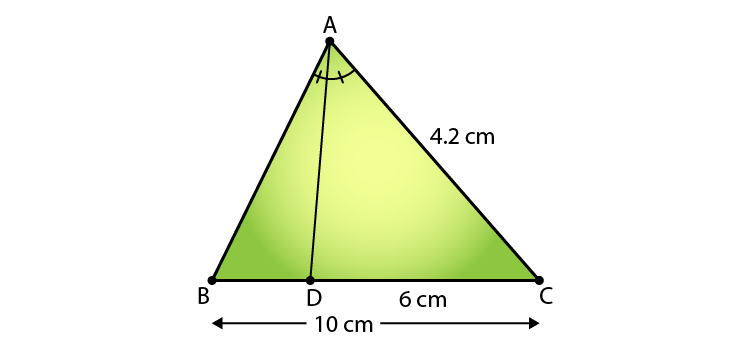Since, AD is the bisector of ∠ A meeting side BC at D in Δ ABC

⇒ AB/ AC = BD/ DC

AB/ 4.2 = BD/ 6

We know that,

BD = BC – DC = 10 – 6 = 4 cm

⇒ AB/ 4.2 = 4/ 6

AB = (2 x 4.2)/ 3

∴ AB = 2.8 cm

(vi) if AB = 5.6 cm, AC = 6 cm, and DC = 3 cm, find BC.

Solution:

Given: Δ ABC and AD bisects ∠A, meeting side BC at D. And AB = 5.6 cm, AC = 6 cm, and DC = 3 cm.

Required to find: BC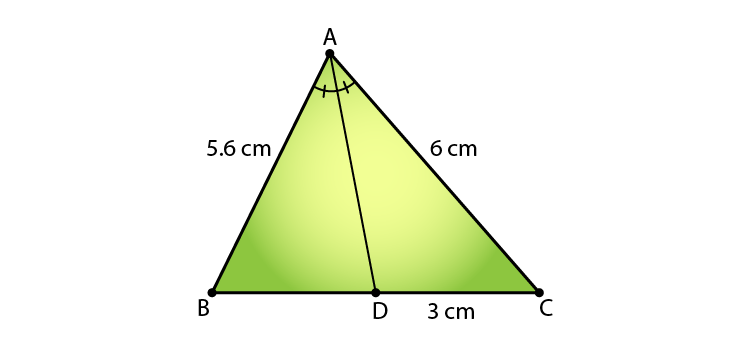Since, AD is the bisector of ∠ A meeting side BC at D in Δ ABC

⇒ AB/ AC = BD/ DC

5.6/ 6 = BD/ 3

BD = 5.6/ 2 = 2.8cm

And, we know that,

BD = BC – DC

2.8 = BC – 3

∴ BC = 5.8 cm

(vii) if AB = 5.6 cm, BC = 6 cm, and BD = 3.2 cm, find AC.

Solution:

Given: Δ ABC and AD bisects ∠A, meeting side BC at D. And AB = 5.6 cm, BC = 6 cm, and BD = 3.2 cm.

Required to find: AC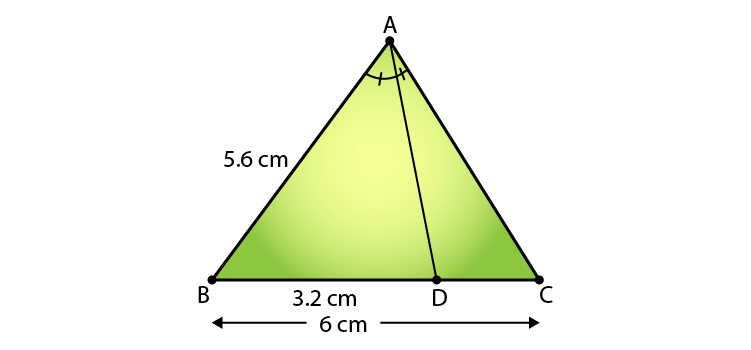Since, AD is the bisector of ∠ A meeting side BC at D in Δ ABC

⇒ AB/ AC = BD/ DC

5.6/ AC = 3.2/ DC

And, we know that

BD = BC – DC

3.2 = 6 – DC

∴ DC = 2.8 cm

⇒ 5.6/ AC = 3.2/ 2.8

AC = (5.6 x 2.8)/ 3.2

∴ AC = 4.9 cm

(viii) if AB = 10 cm, AC = 6 cm, and BC = 12 cm, find BD and DC.

Solution:

Given: Δ ABC and AD bisects ∠A, meeting side BC at D. AB = 10 cm, AC = 6 cm, and BC = 12 cm.

Required to find: DC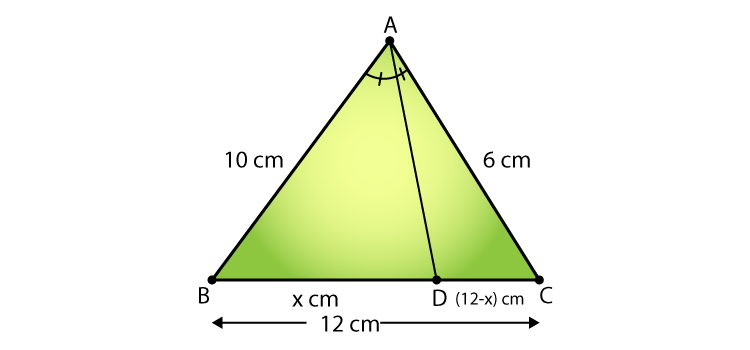Since, AD is the bisector of ∠ A meeting side BC at D in Δ ABC

⇒ AB/ AC = BD/ DC

10/ 6 = BD/ DC …….. (i)

And, we know that

BD = BC – DC = 12 – DC

Let BD = x,

⇒ DC = 12 – x

Thus (i) becomes,

10/ 6 = x/ (12 – x)

5(12 – x) = 3x

60 -5x = 3x

∴ x = 60/8 = 7.5

Hence, DC = 12 – 7.5 = 4.5cm and BD = 7.5 cm

2. In figure 4.57, AE is the bisector of the exterior ∠CAD meeting BC produced in E. If AB = 10 cm, AC = 6 cm, and BC = 12 cm, find CE.

Solution:

Given: AE is the bisector of the exterior CAD and AB = 10 cm, AC = 6 cm, and BC = 12 cm.

Required to find: CE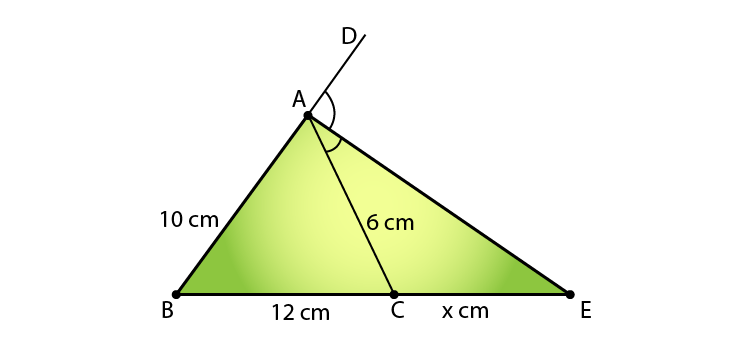Since AE is the bisector of the exterior CAD.

BE / CE = AB / AC

Let’s take CE as x.

So, we have

BE/ CE = AB/ AC

(12+x)/ x = 10/ 6

6x + 72 = 10x

10x – 6x = 72

4x = 72

∴ x = 18

Therefore, CE = 18 cm.

3.  In fig. 4.58, Δ ABC is a triangle such that AB/AC = BD/DC, ∠B=70o, ∠C = 50o, find ∠BAD.

Solution:

Given: Δ ABC such that AB/AC = BD/DC, ∠B = 70o and ∠C = 50o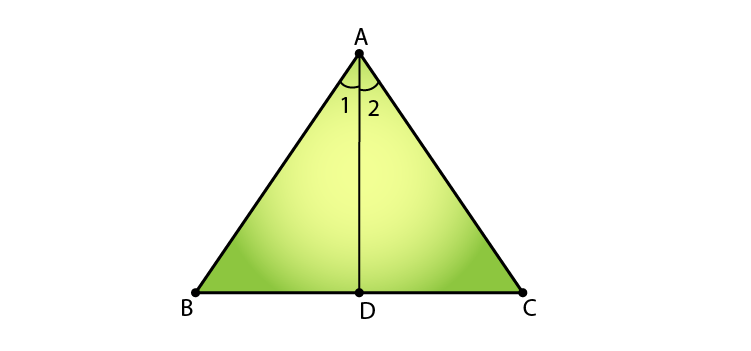We know that,

In ΔABC,

∠A = 180 – (70 + 50) [Angle sum property of a triangle]

= 180 – 120

= 60o

Since,

AB/AC = BD/DC,

AD is the angle bisector of angle ∠A.

Thus,

∠BAD = ∠A/2 = 60/2 = 30o